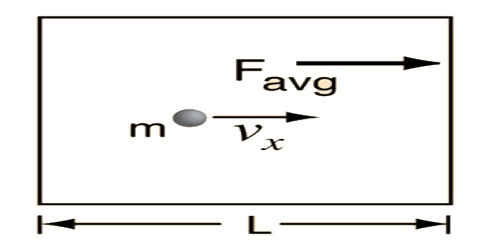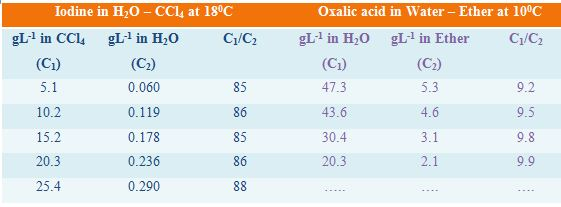# Kinetic Theory of the Distribution Law

Kinetic theory of the distribution law

The distribution law can be derived from the kinetic theory and the rate law. Since the solute is at equilibrium in both the liquids, the rate at which it leaves one phase must be equal to the rate at which it leaves the other phase. i.e., dynamic equilibrium is established. Consequently

Solute (in solvent I) ↔ Solute (in solvent II)

At equilibrium, k1C1 = k2C2

or, C1/C2 = k2/k1 = KD

where, k1 and k2 are rate constants and C1 and C2 are concentrations of the solute in the two phases.

As mentioned the distribution law is valid only when the molecular species of the solute in the two solvents remain the same, i.e., there is no association or dissociation of the solute in any of the solvents. If, however, suitable corrections for such processes are made and only the two solvents are considered the law is found to be fairly obeyed. Deviations are also found to occur when the solute concentration is either very low or very high.

Distribution co-efficient for a particular system changes with temperature as the influence of temperature on the solubility of the solute is different for the two solvents.

In Table, the distribution co-efficients of two solutes in immiscible liquid pairs are given. It can be seen that the values of the distribution co-efficients are reasonably constant showing the validity of the law.

Table: distribution co-efficients